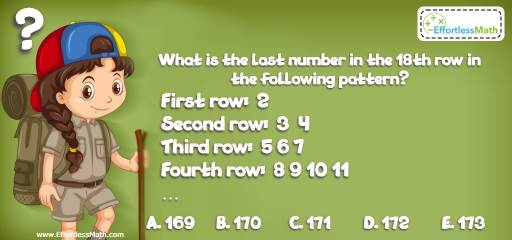# Intelligent Math Puzzle – Challenge 81

Enjoy solving math puzzles and critical thinking challenges? If so, then this challenge is just for you. The answer is also given.## Challenge:

What is the last number in the 18th row in the following pattern?

First row: 2
Second row: 3 4
Third row: 5 6 7
Fourth row: 8 9 10 11

A- 169

B- 170

C- 171

D- 172

E- 173

### The Absolute Best Book to Challenge Your Smart Student!

Each row has one number more than the previous row. The first row has one number, the second row has two numbers, and the 18th row has 18 numbers.
To answer the question, we should find the number of numbers in all 18 rows.
$$1 + 2 + 3 + … + 17 + 18 = \frac{18}{2}(1+18) = 171$$
The first number starts with 2. Therefore, the last number is 172 (171 +1).

The Best Books to Ace Algebra

### What people say about "Intelligent Math Puzzle – Challenge 81 - Effortless Math: We Help Students Learn to LOVE Mathematics"?

No one replied yet.

X
45% OFF

Limited time only!

Save Over 45%

SAVE $40 It was$89.99 now it is \$49.99